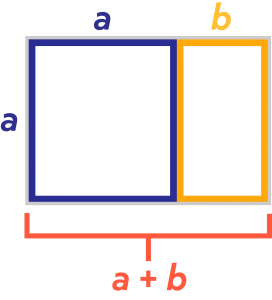# Golden Rectangle Calculator

Go back to Calculators page

This handy calculator will determine the length of either side of the golden rectangle as well as the area, if the other side is known.

## What is the Golden Rectangle?

Seen throughout architecture and nature, the golden rectangle is a rectangle whose sides are in what is called the “golden ratio”.

Recall that a ratio is the relationship between two quantities, represented generally as a fraction. If “a” is the width and “a + b” the length of the rectangle, then the golden ratio is $$\frac{a+b}{a}=\frac{a}{b}$$.  This is what is known as a proportion, which is two ratios set equal to each other.

## How to Calculate the Golden RectangleTo calculate the area of the golden rectangle by hand, simply take the width “a” and multiply by the length “a + b”.

A = a(a + b)

The calculator will quickly check your work for you.

What if the scenario gives you the area but you need to calculate a side?  Suppose you know a + b but not a? Simply divide the area by (a + b) to get the missing side, a.  You can also get b by $$\frac{area}{a}-a$$.

Instead of working everything manually, give the golden rectangle calculator a try for quick and easy results!